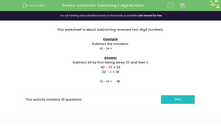Subtraction: Subtracting 2-digit Numbers

In this worksheet, students subtract reversed two-digit numbers and noticing a pattern at the end.Key stage:  KS 2

Curriculum topic:   Number: Addition and Subtraction

Curriculum subtopic:   Use Written Addition/Subtraction to Three Digits

Difficulty level:Worksheet Overview

This worksheet is about subtracting reversed two-digit numbers.

Example

Subtract the numbers.

42 - 24 =

Subtract 24 by first taking away 20 and then 4

42 - 20 = 22

22 - 4 = 18

42 - 24 =
 18

What is EdPlace?

We're your National Curriculum aligned online education content provider helping each child succeed in English, maths and science from year 1 to GCSE. With an EdPlace account you’ll be able to track and measure progress, helping each child achieve their best. We build confidence and attainment by personalising each child’s learning at a level that suits them.

Get started